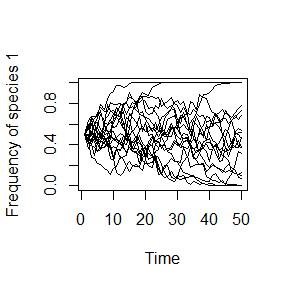## Online Box 2: R code for simulating dynamics with or without constant and frequency-dependent selection in local, two species communities: Figures 6.1, 6.2, 6.3 and 6.6 in the book.

For Fig. 6.1 (neutral model): num.sims = 20, num.years = 50, fit.ratio.avg = 1, freq.dep = 0, init.1 = 0.5*J, J varies among panels

For Fig. 6.2 (constant selection): as for Fig. 6.1 except fit.ratio.avg = 1.1

For Fig. 6.3 (negative frequency-dependent selection): as for Fig. 6.1 except fit.ratio.avg, freq.dep and J vary among panels

For Fig. 6.6 (positive frequency-dependent selection): as for Fig. 6.1 except freq.dep = 0.4, J = 100 and init.1 varies among panels

## specify the number of simulations, the number of years, and a matrix for output
num.sims <- 20
num.years <- 50
freq.1.mat <- matrix(nrow = num.sims, ncol = num.years)

## start a loop for each of num.sims independent simulations
for (j in 1:num.sims) {

## specify parameters and initial conditions
J <- 100
init.1 <- 0.5*J

COM <- vector(length = J)
COM[1:init.1] <- 1; COM[(init.1+1):J] <- 2
year <- 2

fit.ratio.avg <- 1
freq.dep <- 0

## record data (frequency of species 1) for year 1
freq.1.mat[j,1] <- sum(COM==1)/J

## run simulation
for (i in 1:(J*(num.years-1))) {

freq.1 <- sum(COM==1)/J; freq.2 <- 1 - freq.1
fit.ratio <- exp(freq.dep*(freq.1-0.5) + log(fit.ratio.avg))
Pr.1 <- fit.ratio*freq.1/(fit.ratio*freq.1 + freq.2)
COM[ceiling(J*runif(1))] <- sample(c(1,2), 1, prob=c(Pr.1,1-Pr.1))

## record data
if (i %% J == 0) {
freq.1.mat[j,year] <- sum(COM==1)/J
year <- year + 1
}
}
}

## graph the results
plot(1:num.years, freq.1.mat[1,], type="l", xlab="Time",
ylab="Frequency of species 1", ylim=c(0,1))
for (i in 2:(num.sims-1)) {
lines(1:num.years,freq.1.mat[i,], type="l", ylim=c(0,1))
}### Description of the code. Explanations are provided here for parts of the code that have been modified from Box 6.1 (i.e., parts of the already explained in Box 6.1 are not repeated here)

1. As in Box 6.1, we specify the number of years over which each simulation is run: num.years. Since this code runs multiple simulations (as shown in the book figures) we also specify num.sims as the number of simulations to run with a given set of parameters. We also need a matrix freq.1.mat with dimensions numsims * num.years to hold output data, in stead of just a vector.
num.sims <- 20
num.years <- 50
freq.1.mat <- matrix(nrow = num.sims, ncol = num.years)
1. Set values of parameters that determine the strength and nature of selection (or lack thereof). As explained in the book, fit.ratio.avg is the average ratio of species 1’s fitness to species 2’s fitness. When both species’ frequencies are 0.5, fit.ratio (defined inside the simulation loop) is equal to fit.ratio.avg. freq.dep < 0 creates negative frequency-dependent selection, and vice versa for freq.dep > 0. With fit.ratio.avg = 1 and freq.dep = 0, the model is completely neutral.
  fit.ratio.avg <- 1
freq.dep <- 0
1. This is where selection can modify the probability that the individual chosen to reproduce is of one species or the other. The fitness ratio at a given time step, fit.ratio, depends on the selection parameters fit.ratio.avg and freq.dep as well as the species’ frequencies (represented by freq.1), if freq.dep ≠ 0. The probability that the reproducing individual will be species 1, Pr.1, is then determined by fit.ratio and the two species’ frequencies. The form of these equations is explained in the book.
    freq.1 <- sum(COM==1)/J; freq.2 <- 1 - freq.1
fit.ratio <- exp(freq.dep*(freq.1-0.5) + log(fit.ratio.avg))
Pr.1 <- fit.ratio*freq.1/(fit.ratio*freq.1 + freq.2)
1. Graphing the results is done much as in Box 6.1, but now using the lines function to add a separate line for each of the simulations.
plot(1:num.years, freq.1.mat[1,], type="l", xlab="Time",
ylab="Frequency of species 1", ylim=c(0,1))
for (i in 2:(num.sims-1)) {
lines(1:num.years,freq.1.mat[i,], type="l", ylim=c(0,1))